## Chemistry Paper 1 Questions and Answers - KCSE 2020 past papers

Instructions to Candidates

• Non-programmable silent electronic calculators and KNEC mathematical tables may be used
• All working must be clearly shown where necessary.
• Candidates should answer the questions in English.
1. Element A has mass number 40 and 21 neutrons.
1. Write the electron arrangement of element A.  (1 mark)
2. Give the formula of the compound formed when element A reacts with sulphur. (S = 16.0)
(1 mark)
2. Study the setup in Figure 1 and then answer the questions that follow.1. At the end of the experiment, solid B changed from white to blue. Explain. (1 mark)
2. The other product of the burning candle formed a white precipitate with the calcium hydroxide solution. Write an equation for the reaction.  (1 mark)
3. State the role of apparatus C.    (1 mark)
3.
1. State and explain the factors that are considered when collecting a gas by displacement of:
1. air   (1 mark)
2. water.  (1 mark)
2. Other than collecting a gas by displacement of air or water, state another method that can be used to collect a gas. (1 mark)
4.
1. Carbon(II) oxide was passed over 4.1g of heated oxide of copper in a combustion tube until there was no further change. The mass of the final substance was found to be 3.29g
Complete Table 1 and determine the empirical formula of the oxide.
(Cu=64.0; O=16.0)
Table 1
 Element Cu O Mass (g) Number of Moles

Empirical formula                     (2 marks)
2. State the property of carbon(II) oxide that was demonstrated in the experiment. (1 mark)
5.
1. Draw the structural formula of 2-methylbut-2-ene. (1 mark)
2. Bromine water was added to 2-methylbut-2-ene
1. State mark the observation made.  (1 mark)
2. Name the type of the reaction that took place.   (1 mark)
6. Table 2 shows pH values of solutions of compounds D, E. F and G.
Table 2
 Compound D E F G pH value of solution 2 5 7 13

1. State which one of the compounds is likely to be:
1. sodium chloride: (1 mark)
2. ammonium nitrate. (1 mark)
2. Select two compounds that can be used to illustrate the amphoteric nature of an oxide. (1 mark)
3. Give a reason for the answer in (b). (1 mark)
7. Draw a labelled diagram of the setup of apparatus that can be used to electrolyse lead (ll) bromide.   (3 marks)
8.
1. State the difference between a covalent bond and a dative covalent bond.
2. Using dots (•) and crosses (X) to represent electrons, draw a diagram to show the bonding in ammonia.  (1 mark)
3. Using the diagram in (b), state one property that makes ammonia react with hydrogen ion.     1 mark)
9. Figure 2 shows a reaction scheme starting with copper turnings. Study it and answer the questions that follow.1. State the reagent that is added in step 1 (1 mark)
2. ldentify gas H  (1 mark)
3. Write the formula of the complex ion in solution J. (1 mark)
10. When chlorine is bubbled through hot concentrated sodium hydroxide solution, sodium chlorate(V), sodium chloride and water are formed.
1. Write an equation for the reaction. (1 mark)
2. Sodium chlorate(V) and sodium chloride have different solubilities in water. Name a method that can be used to separate the salts.(1 mark)
3. Give one use of sodium chlorate(V). (1 mark)
11. Excess dilute hydrochloric acid was added to an alloy of copper and zinc in a beaker.
1. State the observations made. (2 marks)
2. Excess aqueous sodium hydroxide was added to 2cm3 of the solution obtained in the reaction. Write an ionic equation for the reaction that occured.    (1 mark)
12. Study the information in Table 3 and answer the questions that follow. The elements belong to the same chemical family. (The letters are not actual symbols of the elements)
Table 3
 Element Atomic radius (nm) Ionic radius (nm) Ionisation energy kJ/mol L 0.157 0.095 494 K 0.203 0.133 418 M 0.123 0.060 519 N 0.235 0.169 376

1. Classify the elements as either metals or non-metals. Give a reason. (1 mark)
2.
1. Identify the element which is
1. least reactive     (½mark)
2. most reactive     (½ mark
2. Give a reason for the answer b (i)      (1 mark)
13. Nitrogen(IV) oxide is prepared by heating lead(ll) nitrate.
1. Write an equation for the reaction. (1 mark)
2. At room temperature, nitrogen(IV) oxide exists as an equilibrium mixture with dinitrogen tetraoxide.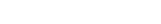Δ is-ve
(brown)       (pale yellow)
State the observation made when the mixture is placed in an ice-bath. Give a reason. (2 marks)
14. Figure 3 shows an energy level diagram for the decomposition of hydrogen peroxide using a catalyst.1. Using the energy values A, B and C, write an expression for
1. ΔH of the reaction    (1 mark)
2. activation energy. (1 mark)
2. On the same axis., sketch a curve that would be obtained if the reaction was carried out without a catalyst.  (1 mark)
15. Sodium carbonate is prepared on large scale by the Solvay process. The equation for the main reaction that takes place in the carbonator is:
NaCl(aq) + NH3(g) + CO2(g)+ H2O(l) → NH4Cl(aq) + NaHCO3(s)
1. Describe how the sodium carbonate is obtained from the products of the carbonator.  (1½ marks)
2. One of the by-products of the Solvay process is calcium chloride. Explain how the calcium chloride is formed in this process. (1½marks)
16. Methane reacts with bromine as shown in the following equation.
CH4(g) + Br2(g) → CH3Br(g) + HBr(g)
Using the bond energies in Table 4, calculate the enthalpy change, ΔH for the reaction.
Table 4
 Bond Bond energy (kJ mol-1) C-H 412 C-Br 276 Br-Br 193 H-Br 366
17. Some compounds such as CFCs and DDT are regarded as environmental pollutants. Give the complete names of:
1. CFCs  (1 mark)
2. DDT (1 mark)
18. Use the information in Table 5 to answer the questions that follow.
Table 5
 Liquid Boiling point (ºC) Miscibility with water Propane 56 Miscible Octane 126 Immiscible Water 100 -

1. State the method that can be used to separate propanone and water.  (1 mark)
2. Describe how a mixture of water and octane can be separated.       (2 marks)
19. 6.2g of phosphorus was reacted with excess oxygen to form phosphorus( V) oxide. Determine the mass of the oxide formed. (0 = 16.0; P 31.0)  (2 marks)
20. Compound V reacts with water as shown in the following equation.
V+H2O → CH3CH2CH2OH
1. Give the structural formula of compound V.      (1 mark)
2. Other than the use of the catalyst, name another condition necessary for this reaction.    (1 mark)
3. This type of reaction is called hydrolysis or hydration. State another name that can be used to describe the reaction.(1 mark)
21. Salts may be classified as soluble or insoluble.
1. Select from the following list a pair of compounds that can be used to prepare a soluble and an insoluble salt.
HNO3, PbNO3)2, KNO3, BaO, NaCl
1. Soluble salt (1 mark)
2. Insoluble salt (1 mark )
2. Describe how a soluble salt is obtained from its solution (1 mark)
22.
1. State one factor that affects the preferential discharge of ions at the cathode (1 mark)
2. Sodium sulphate was electrolysed using inert electrodes. Write the equation for the (b) reaction that takes place at the:
1. cathode: (1 mark)
2. anode (1 mark)
23. Consider the following reaction.
H2S+Cl2 →2HCI+S
Determine the oxidation numbers of chlorine and sulphur in the reactants and products   (2 marks)
 Reactants Products Sulphur Chlorine
24.
1. A volume of sulphur IV) oxide gas diffused from an apparatus in 96 seconds.
Calculate the time taken by an equal volume of carbon(IV) oxide to diffuse under the same conditions. (C = 12.0:    O=16.0; S = 32.0) (1½ mark)
2. The rate of diffusion of neon was found to be 1.45 times faster than that of an equa! volume of gas X at room temperature. Determine the relative formula mass of gas X (Ne = 20.0).  (1½ marks)
25. Complete combustion of one mole of an alkanol, CxHyOH gave four moles of water. (C= 12.0, H = 1.0, O = 16.0)
Determine the:
1. values of x and y
1. X    (1 mark)
2. Y   (1 mark)
2. number of moles of oxygen required for the complete combustion. (1 mark)
26. Radioactive decay of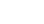gives X,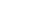and gamma radiation.
1. ldentify X.         (1 mark)
2. Write a nuclear equation for the decay.    (1 mark)
3. The half-life of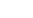is 1.9 years. If after 5.7 years the mass of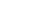was found o be 1.25 g. Determine the initial mass of the radioactive isotope (2 mark)
27. Figure 4 shows part of the structure of a polymer.1. Give the name of the polymer.  (1 mark)
2. Draw the structure of the monomer used.  (1 mark)
3. Give one use of the polymer.   (1 mark)
28. Figure 5 shows variation of number of outermost electrons (a) with atomic mumber of elements in the periodic table.1. Give the values of
1. a1     (1 mark)
2. a3      (1 mark)
2. State why elements with a1 and a2 outermost electrons do not react with each other. (1 mark)## Marking scheme

1. Element A has mass number 40 and 21 neutrons.
1. Write the electron arrangement of element A.  (1 mark)
• No. of electrons;   40−21=19✓½
• Electron arrangement;  2.8.8.1✓½
Accept 2 8 8 1,   2,8,8,1,   2:8:8:1
2. Give the formula of the compound formed when element A reacts with sulphur. (S = 16.0)  (1 mark)
• A2S or SA2 or K2S or SK2
2. Study the setup in Figure 1 and then answer the questions that follow.1. At the end of the experiment, solid B changed from white to blue. Explain. (1 mark)
• Solid B becomes hydrated hence turns from white to blue ✓1
OR
• Burning candles produces water✓½ which combines with solid B to make it hydrated✓½
2. The other product of the burning candle formed a white precipitate with the calcium hydroxide solution. Write an equation for the reaction.  (1 mark)
• CO2(g) + Ca(OH)2(aq) → CaCO3(s) + H2O(l)
(wrong state - penalise symbols ½)
3. State the role of apparatus C.    (1 mark)
• To suck the gaseous product from the system
Accept; to pull/pumping the products/remove the products  Rej; to push
3.
1. State and explain the factors that are considered when collecting a gas by displacement of:
1. air   (1 mark)
• Density of the gas ✓½
• If the gas is lighter than air - upward delivery or downward displacement of air ✓½
OR
• If the gas is denser than air-downward delivery.
(Accept either of the explanation.)
2. water.  (1 mark)
• Solubility in water
• If the gas is insoluble or slightly soluble in water.
(Accept correct explanation without stating)
2. Other than collecting a gas by displacement of air or water, state another method that can be used to collect a gas. (1 mark)
• Gas syringe
• Solidification-SO2
• Condensation
• Freezing
• Collection over mercury
• Liquidification
4.
1. Carbon(II) oxide was passed over 4.1g of heated oxide of copper in a combustion tube until there was no further change. The mass of the final substance was found to be 3.29g
Complete Table 1 and determine the empirical formula of the oxide.
(Cu=64.0; O=16.0)
Table 1
 Element Cu O Mass (g) 3.29 0.81✓½ Number of Moles 3.29    64=0.051✓½ 0.81    16=0.051✓½
Mole ratio             1               1
Empirical formula        CuO             (2 marks)
2. State the property of carbon(II) oxide that was demonstrated in the experiment. (1 mark)
• Reducing agent/Reducing property.
5.
1. Draw the structural formula of 2-methylbut-2-ene. (1 mark)(All bonds must be shown.      Rej. Condensed formula)
2. Bromine water was added to 2-methylbut-2-ene
1. State mark the observation made.  (1 mark)
• Bromine water is decolourised.
• Orange/brown/yellow bromine turns to colourless (Emphasis colourless)
Rej. If initial colour is not original colour of bromine
2. Name the type of the reaction that took place.   (1 mark)
• Addition reaction.   Rej. Halogenation or Bromination process
6. Table 2 shows pH values of solutions of compounds D, E. F and G.
Table 2
 Compound D E F G pH value of solution 2 5 7 13

1. State which one of the compounds is likely to be:
1. sodium chloride: (1 mark)
• F or pH7
2. ammonium nitrate. (1 mark)
• E or pH5
2. Select two compounds that can be used to illustrate the amphoteric nature of an oxide. (1 mark)
• D and G or 2 and 13 ✓½each  Marked independently
3. Give a reason for the answer in (b). (1 mark)
• Amphoteric oxides react with both strong acids/acids and alkalines
OR
• Amphoteric oxides behave as acids or bases.
7. Draw a labelled diagram of the setup of apparatus that can be used to electrolyse lead (ll) bromide.   (3 marks)Workability
• Electrodes are above the electrolyte
• Cells
• Heat
• Anodes and Cathodes correctly labelled
8.
1. State the difference between a covalent bond and a dative covalent bond.
• In covalent shared electrons are contributed by both species/atoms while in dative, the shared electrons are from on of the atoms or species.
2. Using dots (•) and crosses (X) to represent electrons, draw a diagram to show the bonding in ammonia.  (1 mark)(Award ½ a mark if a • or X is used)
3. Using the diagram in (b), state one property that makes ammonia react with hydrogen ion.     1 mark)
• Nitrogen in Ammonia contain a lone pair of electrons.
OR
• Presence of lone pair of electrons/ unshared pair of e-
9. Figure 2 shows a reaction scheme starting with copper turnings. Study it and answer the questions that follow.1. State the reagent that is added in step 1 (1 mark)
• Conc. Sulphuric(VI) acid, Conc. H2SO4 or Sulphuric(VI) acid
Rej. Dilute or H2SO4(aq)
2. ldentify gas H  (1 mark)
•  SO2 or sulphur (IV) Oxide.
3. Write the formula of the complex ion in solution J. (1 mark)
• (Cu(NH3)4)2+
10. When chlorine is bubbled through hot concentrated sodium hydroxide solution, sodium chlorate(V), sodium chloride and water are formed.
1. Write an equation for the reaction. (1 mark)
3Cl2(g) + 6NaOH(aq) → NaClO3(aq) + 5 NaCl(aq) + 3H2O(l)
2. Sodium chlorate(V) and sodium chloride have different solubilities in water. Name a method that can be used to separate the salts.(1 mark)
• Fractional crystallisation.
3. Give one use of sodium chlorate(V). (1 mark)
• Herbicides
• Manufacture of dyes
• Bleaching agent
• Treatment of sewage
• In fireworks and explosives/matches.
11. Excess dilute hydrochloric acid was added to an alloy of copper and zinc in a beaker.
1. State the observations made. (2 marks)
• Fizzing/Effervescence/production of gas bubbles✓1
• Colourless solution formed ✓½
• Brown residue/solid ✓½
2. Excess aqueous sodium hydroxide was added to 2cm3 of the solution obtained in the reaction. Write an ionic equation for the reaction that occured.    (1 mark)
• Zn2+(aq) + 4OH(aq) → (Zn(OH)4)2−(aq)
OR
• Zn(OH)2)(s) + 2OH(aq) → (Zn(OH)4)2−(aq)
12. Study the information in Table 3 and answer the questions that follow. The elements belong to the same chemical family. (The letters are not actual symbols of the elements)
Table 3
 Element Atomic radius (nm) Ionic radius (nm) Ionisation energy kJ/mol L 0.157 0.095 494 K 0.203 0.133 418 M 0.123 0.060 519 N 0.235 0.169 376

1. Classify the elements as either metals or non-metals. Give a reason. (1 mark)
• Metals ✓½ Ionic radius✓½ is smaller than corresponding atomic radius/ vice versa
2.
1. Identify the element which is
1. least reactive  ✓½
2. most reactive  N  ✓½
2. Give a reason for the answer b (i)      (1 mark)
• The more the ionization energy, the less the reactive or the less the ionization energy the more the reaction OR use atomic radius to explain.
(Accept a lot of heat is required/less heat is required)
13. Nitrogen(IV) oxide is prepared by heating lead(ll) nitrate.
1. Write an equation for the reaction. (1 mark)
• 2Pb(NO3)2(s)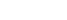2PbO(s) + 4NO2(g) + O2(g)
2. At room temperature, nitrogen(IV) oxide exists as an equilibrium mixture with dinitrogen tetraoxide.Δ is-ve
(brown)       (pale yellow)
State the observation made when the mixture is placed in an ice-bath. Give a reason. (2 marks)
• Mixture turns yellow ✓1/yellow colour intensifies
• Decrease in temperature,forward is favoured ✓½ /more N2O4 is formed since the reaction is exothermic✓½
14. Figure 3 shows an energy level diagram for the decomposition of hydrogen peroxide using a catalyst.1. Using the energy values A, B and C, write an expression for
1. ΔH of the reaction    (1 mark)
• ΔH = A−B ✓1
2. activation energy. (1 mark)
• EA = C−B✓1
Or Diagram award ½
2. On the same axis., sketch a curve that would be obtained if the reaction was carried out without a catalyst.  (1 mark)15. Sodium carbonate is prepared on large scale by the Solvay process. The equation for the main reaction that takes place in the carbonator is:
NaCl(aq) + NH3(g) + CO2(g)+ H2O(l) → NH4Cl(aq) + NaHCO3(s)
1. Describe how the sodium carbonate is obtained from the products of the carbonator.  (1½ marks)
• The products are filtered ✓½ to obtain NaHCO3 as a residue.
• The residue is heated to obtain NaHCO✓1
OR
• The products are filtered then dried ✓1
2. One of the by-products of the Solvay process is calcium chloride. Explain how the calcium chloride is formed in this process. (1½marks)
• Calcium carbonate decompose to form CaO and CO2
• CaO/Ca(OH)2 reacts with water and then NH4Cl to form CaCl2
OR Use of equations correctly
16. Methane reacts with bromine as shown in the following equation.
CH4(g) + Br2(g) → CH3Br(g) + HBr(g)
Using the bond energies in Table 4, calculate the enthalpy change, ΔH for the reaction.
Table 4
 Bond Bond energy (kJ mol-1) C-H 412 C-Br 276 Br-Br 193 H-Br 366

Bonds broken                        Bonds formed
C-H                                        C-Br
Br-Br                                      H-Br
Energy absorbed                    Energy released
412 + 193                              276 + 366
=605 kJ                                  =642kJ
ΔH = 605 - 642
= −37kJ/mol
OR
Bonds broken                         Bonds formed
(4 × 412) + 193                     (3 ×−412) 1 −276 +−366
=+1841                                   =−1878
ΔH= 1841+−1878
=−37kJ/mol
17.  Some compounds such as CFCs and DDT are regarded as environmental pollutants. Give the complete names of:
1. CFCs  (1 mark)
• Chlorofluorocarbons
2. DDT (1 mark)
• Dichlorodiphenyltrichloroethane.
18. Use the information in Table 5 to answer the questions that follow.
Table 5
 Liquid Boiling point (ºC) Miscibility with water Propane 56 Miscible Octane 126 Immiscible Water 100 -
1. State the method that can be used to separate propanone and water.  (1 mark)
• Fractional distillation/ distillation
2. Describe how a mixture of water and octane can be separated.       (2 marks)
• Place the mixture on a separating funnel/ dropping funnel/ burette
• Run down the bottom layer.
OR
• Use of a dropper
• Suck the top layer
• Suck the interphase and discard it
(Penalise ½ if interphase is not discarded)
19. 6.2g of phosphorus was reacted with excess oxygen to form phosphorus( V) oxide. Determine the mass of the oxide formed. (0 = 16.0; P 31.0)  (2 marks)
4P(s) + 5O2(g) → 2P2O5(g)
Moles of P =  6.2   =0.2✓½
31
Moles of P2O5 = ½ × 0.2 = 0.1moles
R.F.M of P2O5 = (31× 2) + ( 16×5) =142
Mass of P2O5 = 142 × 0.1 = 14.2g
20. Compound V reacts with water as shown in the following equation.
V+H2O → CH3CH2CH2OH
1. Give the structural formula of compound V.      (1 mark)
CH3CH=CH2 or CH3CHCH2 or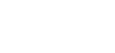2. Other than the use of the catalyst, name another condition necessary for this reaction.    (1 mark)
• Heat or pressure of 60-70 atmosphere -Temp of 250-300°C.
Rej warming
3. This type of reaction is called hydrolysis or hydration. State another name that can be used to describe the reaction.(1 mark)
21. Salts may be classified as soluble or insoluble.
1. Select from the following list a pair of compounds that can be used to prepare a soluble and an insoluble salt.
HNO3, Pb(NO3)2, KNO3, BaO, NaCl
1. Soluble salt (1 mark)
• HNO3 or BaO
2. Insoluble salt (1 mark )
• Pb(NO3)2 and NaCl
2. Describe how a soluble salt is obtained from its solution (1 mark)
• Heat/boil to evaporate ✓½ the mixture to saturation
• Allow to cool✓½
• Filter the residue.
22.
1. State one factor that affects the preferential discharge of ions at the cathode (1 mark)
• Nature of the electrolyte
• Concentration
• Position of the element in the reactivity series
• Position of the electrochemical series
2. Sodium sulphate was electrolysed using inert electrodes. Write the equation for the (b) reaction that takes place at the:
1. cathode: (1 mark)
• 2H+(aq) + 2e→ H2(g)
OR
• 4H+(aq) + 4e → 2H2(g)
2. anode (1 mark)
• 4OH(aq) →2H2O(l) + O2(g) + 4e
23. Consider the following reaction.
H2S+Cl2 →2HCI+S
Determine the oxidation numbers of chlorine and sulphur in the reactants and products   (2 marks)
 Reactants Products Sulphur −2✓½ 0✓½ Chlorine 0✓½ −1✓½
24.
1. A volume of sulphur IV) oxide gas diffused from an apparatus in 96 seconds.
Calculate the time taken by an equal volume of carbon(IV) oxide to diffuse under the same conditions. (C = 12.0:    O=16.0; S = 32.0) (1½ mark)
RMM of SO2 =64✓½
RMM of CO2 = 44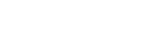tCO2 = 79.605  ✓½
2. The rate of diffusion of neon was found to be 1.45 times faster than that of an equa! volume of gas X at room temperature. Determine the relative formula mass of gas X (Ne = 20.0).  (1½ marks)
RNe =1.45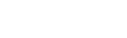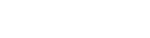MX =2.1025 × 20 ✓½
=42.05
=42 ✓½
25. Complete combustion of one mole of an alkanol, CxHyOH gave four moles of water. (C= 12.0, H = 1.0, O = 16.0)
Determine the:  CxHyOH + O2 → CO2 +4H2O
1. values of x and y
1. X = 3  (1 mark)
2. Y =7   (1 mark)
2. number of moles of oxygen required for the complete combustion. (1 mark)
• 3 moles
26. Radioactive decay of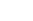gives X,and gamma radiation.
1. ldentify X.         (1 mark)
2. Write a nuclear equation for the decay.    (1 mark)
3. The half-life of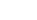is 1.9 years. If after 5.7 years the mass of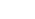was found to be 1.25 g. Determine the initial mass of the radioactive isotope (2 marks)
No. of half life = 5.7  = 3
1.9
1st                   2nd                   3rd
1.25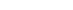2.55.010.0
=10.0g
27. Figure 4 shows part of the structure of a polymer.1. Give the name of the polymer.  (1 mark)
• polyphenylethene or polystyrene
2. Draw the structure of the monomer used.  (1 mark)3. Give one use of the polymer.   (1 mark)
• Packaging materials
• Insulator
• Ceiling boards
28. Figure 5 shows variation of number of outermost electrons (a) with atomic number of elements in the periodic table.1. Give the values of
1. a1 1   (1 mark)
2. a3 8      (1 mark)
2. State why elements with a1 and a2 outermost electrons do not react with each other. (1 mark)
• Have a tendency to loose electrons.
• Both form positive ions.
• Both of them are metals.

• ✔ To read offline at any time.
• ✔ To Print at your convenience
• ✔ Share Easily with Friends / Students